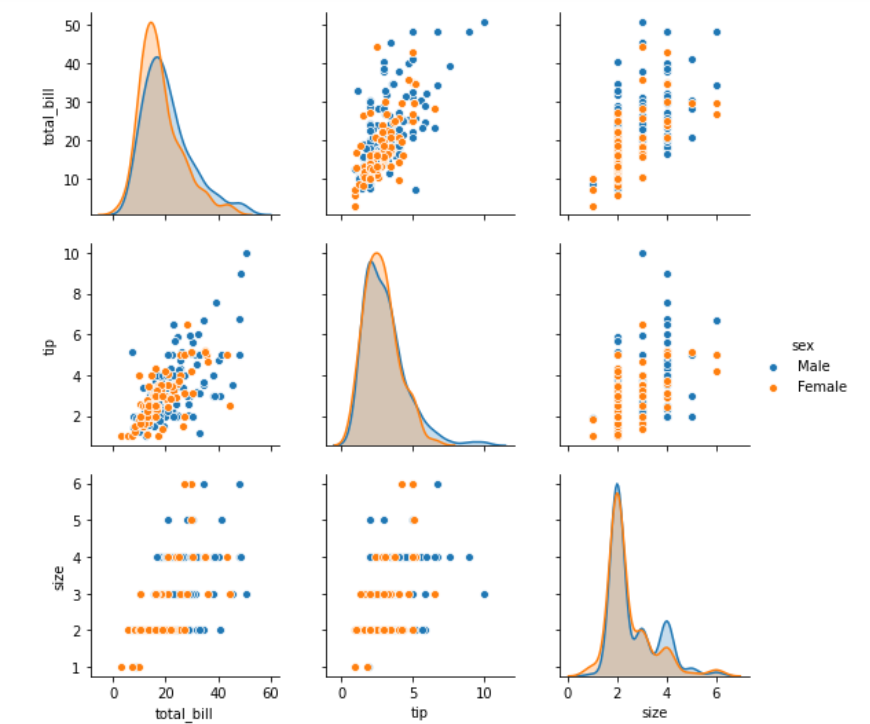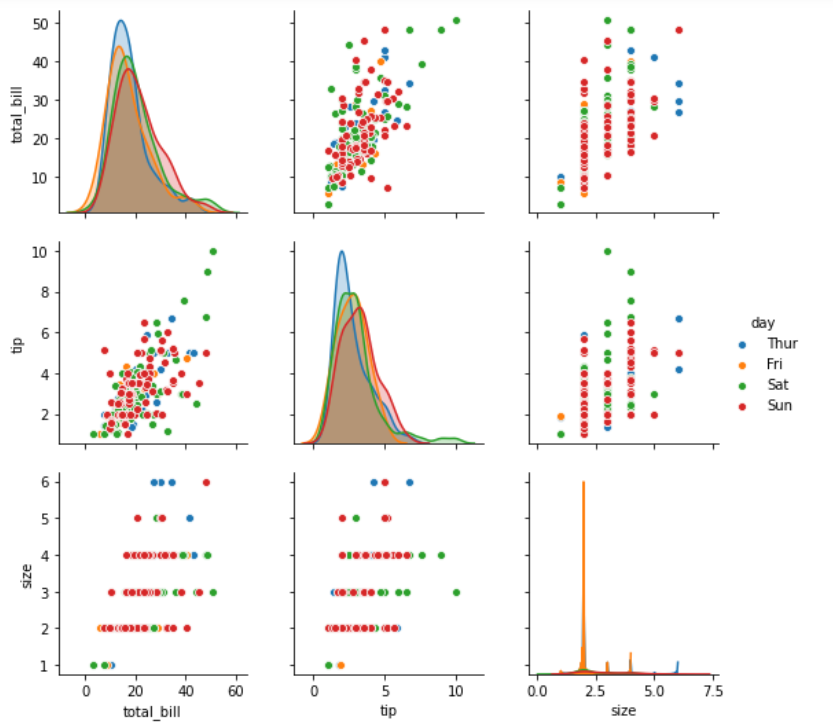# Python – seaborn.pairplot() method

• Difficulty Level : Medium
• Last Updated : 15 Jul, 2020

#### Prerequisite: Seaborn Programming Basics

Seaborn is a Python data visualization library based on matplotlib. It provides a high-level interface for drawing attractive and informative statistical graphics. Seaborn helps resolve the two major problems faced by Matplotlib; the problems are ?

• Default Matplotlib parameters
• Working with data frames

As Seaborn compliments and extends Matplotlib, the learning curve is quite gradual. If you know Matplotlib, you are already half way through Seaborn.

### seaborn.pairplot() :

To plot multiple pairwise bivariate distributions in a dataset, you can use the pairplot() function. This shows the relationship for (n, 2) combination of variable in a DataFrame as a matrix of plots and the diagonal plots are the univariate plots.

```                        seaborn.pairplot( data, \*\*kwargs )

```

Seaborn.pairplot uses many arguments as input, main of which are described below in form of table:

Below is the implementation of above method:

## Python3

 `# importing packages``import` `seaborn``import` `matplotlib.pyplot as plt`` ` `############# Main Section ############``# loading dataset using seaborn``df ``=` `seaborn.load_dataset(``'tips'``)``# pairplot with hue sex``seaborn.pairplot(df, hue ``=``'sex'``)``# to show``plt.show()`` ` `# This code is contributed by Deepanshu Rustagi.`

#### Output :## Python3

 `# importing packages``import` `seaborn``import` `matplotlib.pyplot as plt`` ` `############# Main Section ############``# loading dataset using seaborn``df ``=` `seaborn.load_dataset(``'tips'``)``# pairplot with hue day``seaborn.pairplot(df, hue ``=``'day'``)``# to show``plt.show()`` ` `# This code is contributed by Deepanshu Rustagi.`

#### Output :My Personal Notes arrow_drop_up